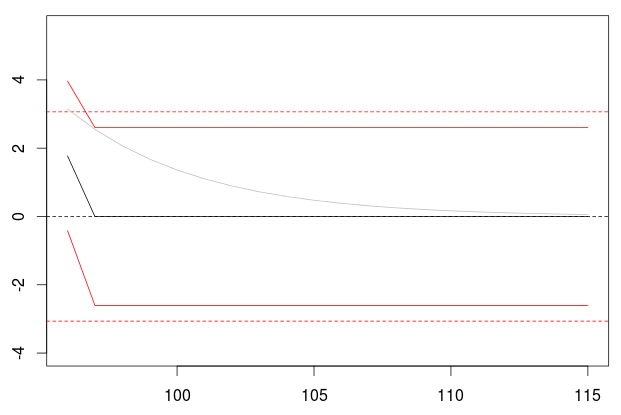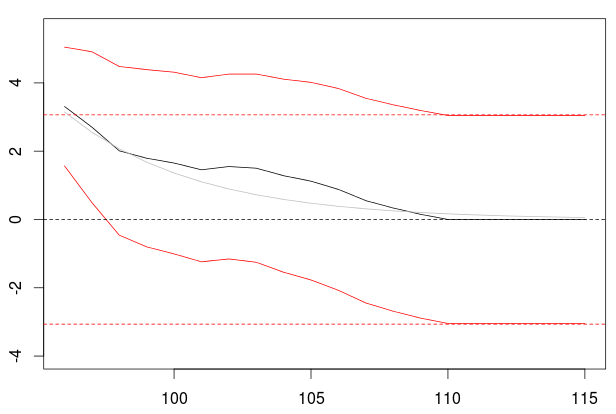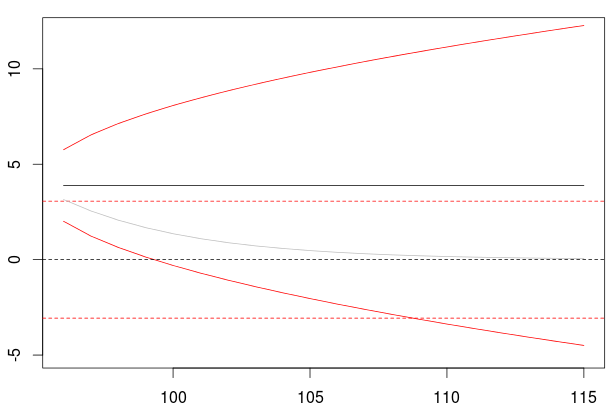Want to share your content on R-bloggers? click here if you have a blog, or here if you don't.

In our time series class this morning, I was discussing forecasts with ARIMA Models. Consider some simple stationnary AR(1) simulated time series

```> n=95
> set.seed(1)
> E=rnorm(n)
> X=rep(0,n)
> phi=.85
> for(t in 2:n) X[t]=phi*X[t-1]+E[t]
> plot(X,type="l")```If we fit an AR(1) model,

```> model=arima(X,order=c(1,0,0),
+             include.mean = FALSE)
> plot(P\$pred)
> lines(P\$pred+2*P\$se,col="red")
> lines(P\$pred-2*P\$se,col="red")
> abline(h=0,lty=2)
> abline(h=2*P\$se,lty=2,col="red")
> abline(h=-2*P\$se,lty=2,col="red")```we observe the exponential decay of the forecast towards 0, and the increasing confidence interval (where the variance increases, from the variance of the white noise to the variance of the stationnary time series). Plain lines are conditional forecast (given our latest observation, since the AR(1) is a first order Markov process), and dotted lines are unconditional. Let us store some values, to use them as benchmark

```> s=P\$se
> y=P\$pred```

If we fit a MA(1) model

```> model=arima(X,order=c(0,0,1),
+             include.mean = FALSE)
> plot(P\$pred)
> lines(P\$pred+2*P\$se,col="red")
> lines(P\$pred-2*P\$se,col="red")
> abline(h=0,lty=2)
> abline(h=2*s,lty=2,col="red")
> abline(h=-2*s,lty=2,col="red")
> lines(y,col="grey")```after two lags, the forecast is null, and the (conditional) variance remains constant. But if we consider a moving average process with a longer order,

```> model=arima(X,order=c(0,0,14),
+             include.mean = FALSE)
> plot(P\$pred)
> lines(P\$pred+2*P\$se,col="red")
> lines(P\$pred-2*P\$se,col="red")
> abline(h=0,lty=2)
> abline(h=2*s,lty=2,col="red")
> abline(h=-2*s,lty=2,col="red")
> lines(y,col="grey")```we get an output that can be compared with the AR(1) processes. Which makes sense since our AR(1) process can also be seen as a MA(∞), with infinite order.

But if we think that our time series is not stationary, an we fit an integrated model

```> model=arima(X,order=c(0,1,0),
+             include.mean = FALSE)
> plot(P\$pred)
> lines(P\$pred+2*P\$se,col="red")
> lines(P\$pred-2*P\$se,col="red")
> abline(h=0,lty=2)
> abline(h=2*s,lty=2,col="red")
> abline(h=-2*s,lty=2,col="red")
> lines(y,col="grey")```we observe the (standard) martingale property: the forecast is flat, and the confidence interval keeps increasing, and actually, the variance increases towards infinity (at a linear rate). So one should be very careful when differentiation a time series… it will have a huge impact on the forecasts….